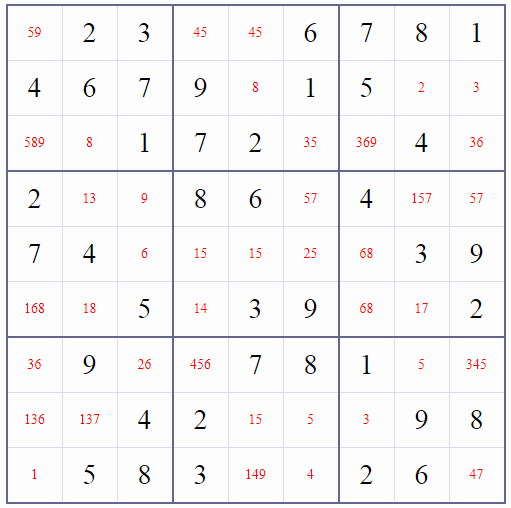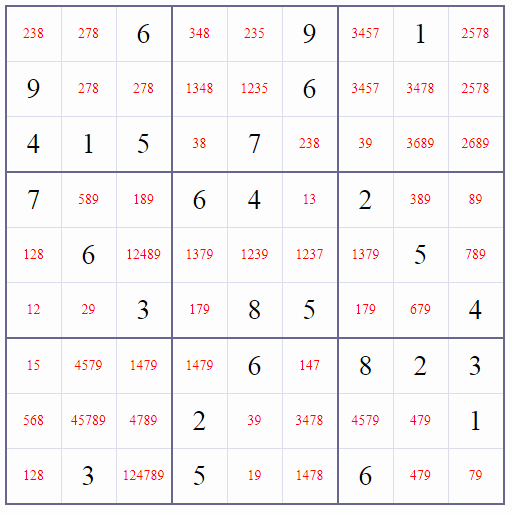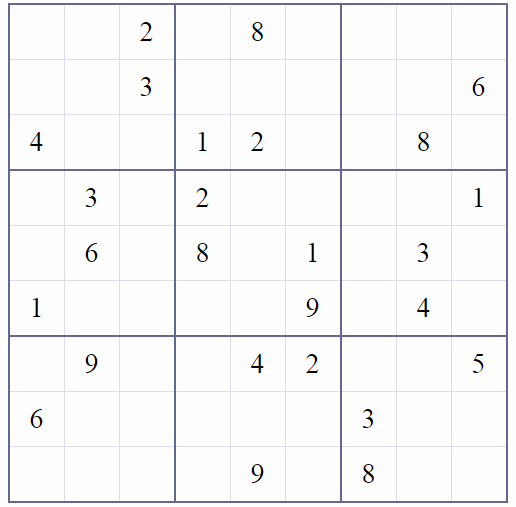Chat

# How Do I Measure the Difficulty Level of a Sudoku Puzzle?

A number game (Sudoku) is a puzzle in which missing numbers are to be filled into a 9 by 9 grid of squares which are subdivided into 3 by 3 boxes so that every row, every column, and every box contains the numbers 1 through 9.

To explain why some Sudoku puzzles are easier to solve while others are more challenging. Let us look at two Sudoku puzzles and judge which one is more difficult at the start of solving it.

For this Sudoku, if you write down their candidate values and use OneChoice method, and you can immediately solve 11 empty squares.Let us take a look at another one. How many empty squares can be inferred immediately using OneChoice method? (Answer: 0)You will have to use Elimination and other advanced solving Sudoku methods to refine the candiate values of empty squares.

Therefore, at least in the first step, the first Sudoku is easier than the second Sudoku.

Usually, an easy Sudoku can be solved by using OneChoice and Elimination.

OneChoice: An empty square has to be a certain number because all other numbers already appear in its row/column/box.

Elimination: An untaken number in a row/column/box must be in a certain empty square because all other empty squares can not be this number.

A medium Sudoku puzzle needs to be solved using logical reasoning, such as Interaction, Subset, X-Wing, XY-Wing, XYZ-Wing. These methods, instead of deciding which untaken must goes to an empty square in a row/column/box, or deciding which empty square in a row/column/box has to be a certain number, refines the candidate numbers of each empty square.

A hard Sudoku, you will have to use at least one guess (if a guess of a number in an empty square causes conflict, we can eliminate this number from this empty square’s candidate numbers. This is called ConflictElimination).

An evil Sudoku will generally involve either a significant number of simple guesses or some number of more complicated guesses (Exhaustive Search).

If you made a Sudoku puzzle, an easy way to rate a Sudoku puzzle based on the methods that a Sudoku lover have to use based on the assumption that an advanced method is only used when easier methods do not work. Usually, if an advanced method is used, the average effort for all Sudoku solver would be much higher because some of Sudoku solvers would be stuck because they do not know how to use this advanced method.

The best way is to let Sudoku lovers rate the difficulty level of a Sudoku puzzle. How do you rate this Sudoku puzzle?